Will you get all the answers correct?

# Statistics (Mean, Mode, Median and Range)

This Math quiz is called 'Statistics (Mean, Mode, Median and Range)' and it has been written by teachers to help you if you are studying the subject at middle school. Playing educational quizzes is a fabulous way to learn if you are in the 6th, 7th or 8th grade - aged 11 to 14.

It costs only \$12.50 per month to play this quiz and over 3,500 others that help you with your school work. You can subscribe on the page at Join Us

Data is a collection of information, arranged in such a way that it can be analyzed. This middle school Math quiz will explore some of the common tools that can be used to analyze data.

In very general terms, data can be split into two types – qualitative data is descriptive (i.e. uses words), and quantitative data is numerical. In-depth analysis of descriptive data can be quite hard to do, whereas it is easy and quick to gain some understanding of numerical data.

The two key questions when analyzing data are: where is it generally located? From this, we look at the different types of averages.

The second question is how far spread out is it? This gives us the range.

It is important to know the three types of averages, and when it is appropriate to use each one. The MODE is the MOst common. If you are studying French, Spanish or Italian at middle school what is the word that means fashion? It will be similar to la mode, moda. So the mode is the most fashionable number in the list.

The MEDIAN is the MIDdle number, when the numbers are arranged in size order. If there is an odd number of numbers, then there will only be one middle number. However, if there is an even number of numbers in the list, then you take the sum of the two middle numbers, and divide by 2. Don’t panic if the answer is not in the original list, it is still correct!

The MEAN is the hardest, or MEANEST to calculate. This is where you add up all the numbers, then divide by the number of numbers in the list. When the media talk about an ‘average’, this is the one they generally mean.

The RANGE is simply the difference between the largest and the smallest value.

Question 1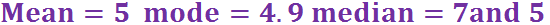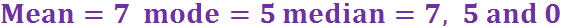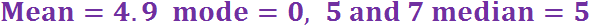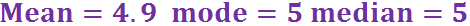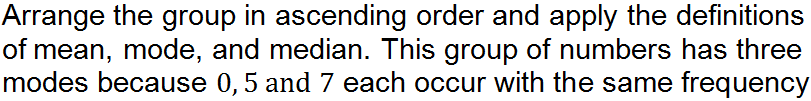Question 2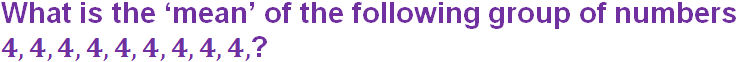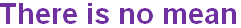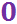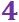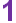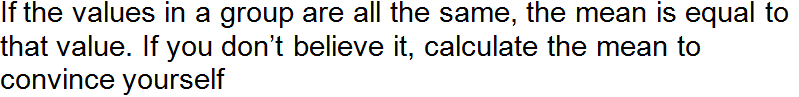Question 3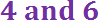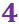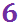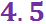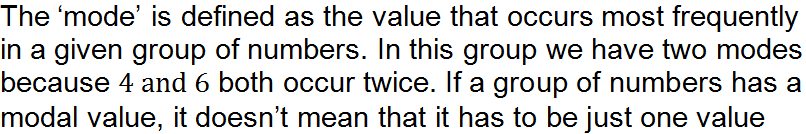Question 4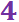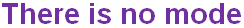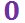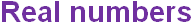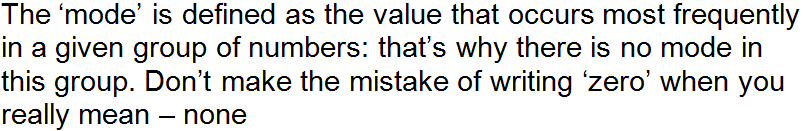Question 5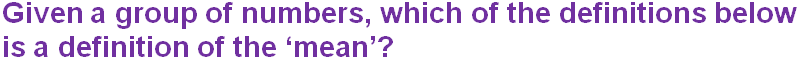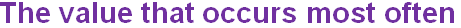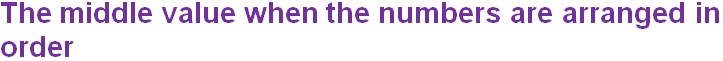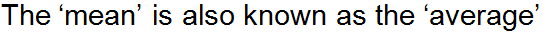Question 6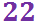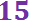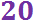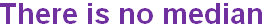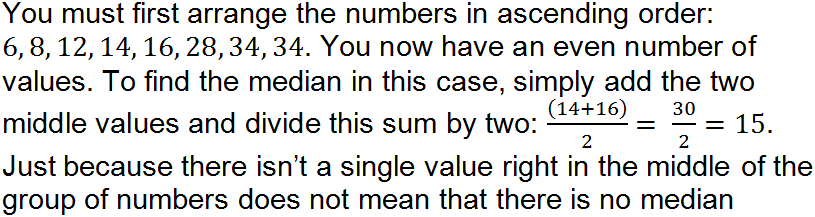Question 7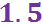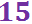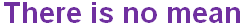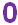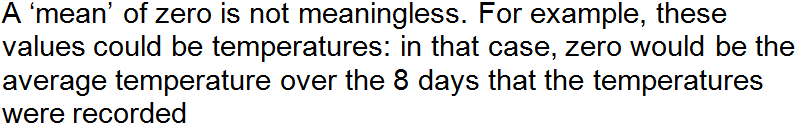Question 8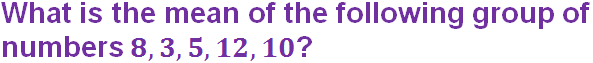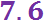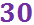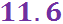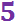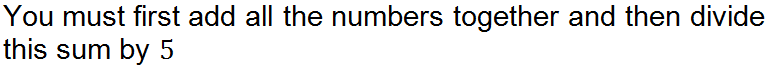Question 9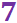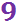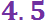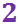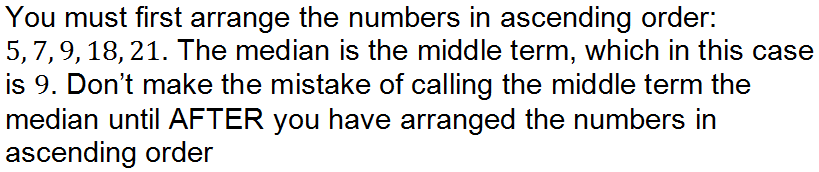Question 10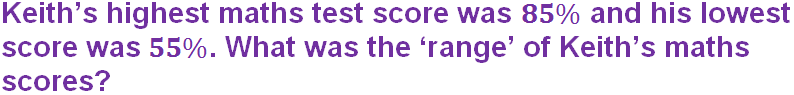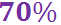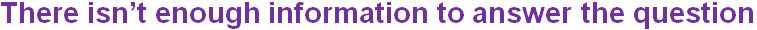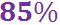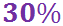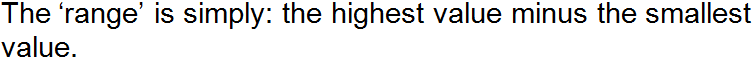Author:  Frank Evans